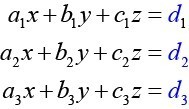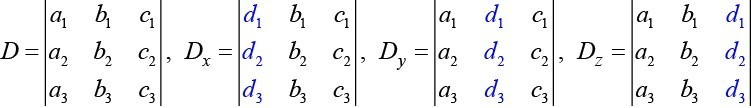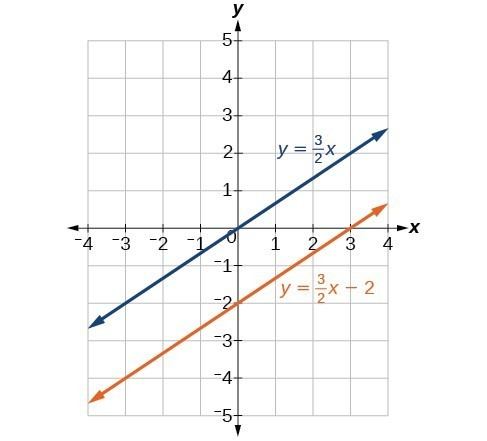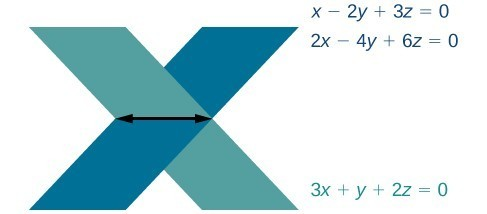## Evaluating the Determinant of a 3 × 3 Matrix

Finding the determinant of a 2×2 matrix is straightforward, but finding the determinant of a 3×3 matrix is more complicated. One method is to augment the 3×3 matrix with a repetition of the first two columns, giving a 3×5 matrix. Then we calculate the sum of the products of entries down each of the three diagonals (upper left to lower right), and subtract the products of entries up each of the three diagonals (lower left to upper right). This is more easily understood with a visual and an example.

Find the determinant of the 3×3 matrix.

$A=\left[\begin{array}{ccc}{a}_{1}& {b}_{1}& {c}_{1}\\ {a}_{2}& {b}_{2}& {c}_{2}\\ {a}_{3}& {b}_{3}& {c}_{3}\end{array}\right]$
1. Augment $A$ with the first two columns.
$\mathrm{det}\left(A\right)=|\begin{array}{ccc}{a}_{1}& {b}_{1}& {c}_{1}\\ {a}_{2}& {b}_{2}& {c}_{2}\\ {a}_{3}& {b}_{3}& {c}_{3}\end{array}|\begin{array}{c}{a}_{1}\\ {a}_{2}\\ {a}_{3}\end{array}\begin{array}{c}{b}_{1}\\ {b}_{2}\\ {b}_{3}\end{array}|$
2. From upper left to lower right: Multiply the entries down the first diagonal. Add the result to the product of entries down the second diagonal. Add this result to the product of the entries down the third diagonal.
3. From lower left to upper right: Subtract the product of entries up the first diagonal. From this result subtract the product of entries up the second diagonal. From this result, subtract the product of entries up the third diagonal.Figure 2

The algebra is as follows:

$|A|={a}_{1}{b}_{2}{c}_{3}+{b}_{1}{c}_{2}{a}_{3}+{c}_{1}{a}_{2}{b}_{3}-{a}_{3}{b}_{2}{c}_{1}-{b}_{3}{c}_{2}{a}_{1}-{c}_{3}{a}_{2}{b}_{1}$

### Example 3: Finding the Determinant of a 3 × 3 Matrix

Find the determinant of the 3 × 3 matrix given

$A=\left[\begin{array}{ccc}0& 2& 1\\ 3& -1& 1\\ 4& 0& 1\end{array}\right]$

### Solution

Augment the matrix with the first two columns and then follow the formula. Thus,

$\begin{array}{l}|A|=|\begin{array}{ccc}0& 2& 1\\ 3& -1& 1\\ 4& 0& 1\end{array}|\begin{array}{c}0\\ 3\\ 4\end{array}\begin{array}{c}2\\ -1\\ 0\end{array}|\hfill \\ =0\left(-1\right)\left(1\right)+2\left(1\right)\left(4\right)+1\left(3\right)\left(0\right)-4\left(-1\right)\left(1\right)-0\left(1\right)\left(0\right)-1\left(3\right)\left(2\right)\hfill \\ =0+8+0+4 - 0-6\hfill \\ =6\hfill \end{array}$

### Try It 2

Find the determinant of the 3 × 3 matrix.

$\mathrm{det}\left(A\right)=|\begin{array}{ccc}1& -3& 7\\ 1& 1& 1\\ 1& -2& 3\end{array}|$

### Can we use the same method to find the determinant of a larger matrix?

No, this method only works for $2\text{ }\times \text{ }2$ and $\text{3}\text{ }\times \text{ }3$ matrices. For larger matrices it is best to use a graphing utility or computer software.

## Using Cramer’s Rule to Solve a System of Three Equations in Three Variables

Now that we can find the determinant of a 3 × 3 matrix, we can apply Cramer’s Rule to solve a system of three equations in three variables. Cramer’s Rule is straightforward, following a pattern consistent with Cramer’s Rule for 2 × 2 matrices. As the order of the matrix increases to 3 × 3, however, there are many more calculations required.

When we calculate the determinant to be zero, Cramer’s Rule gives no indication as to whether the system has no solution or an infinite number of solutions. To find out, we have to perform elimination on the system.

Consider a 3 × 3 system of equations.Figure 3

$x=\frac{{D}_{x}}{D},y=\frac{{D}_{y}}{D},z=\frac{{D}_{z}}{D},D\ne 0$

whereFigure 4

If we are writing the determinant ${D}_{x}$, we replace the $x$ column with the constant column. If we are writing the determinant ${D}_{y}$, we replace the $y$ column with the constant column. If we are writing the determinant ${D}_{z}$, we replace the $z$ column with the constant column. Always check the answer.

### Example 4: Solving a 3 × 3 System Using Cramer’s Rule

Find the solution to the given 3 × 3 system using Cramer’s Rule.

$\begin{array}{c}x+y-z=6\\ 3x - 2y+z=-5\\ x+3y - 2z=14\end{array}$

### Solution

Use Cramer’s Rule.

$D=|\begin{array}{ccc}1& 1& -1\\ 3& -2& 1\\ 1& 3& -2\end{array}|,{D}_{x}=|\begin{array}{ccc}6& 1& -1\\ -5& -2& 1\\ 14& 3& -2\end{array}|,{D}_{y}=|\begin{array}{ccc}1& 6& -1\\ 3& -5& 1\\ 1& 14& -2\end{array}|,{D}_{z}=|\begin{array}{ccc}1& 1& 6\\ 3& -2& -5\\ 1& 3& 14\end{array}|$

Then,

$\begin{array}{l}x=\frac{{D}_{x}}{D}=\frac{-3}{-3}=1\hfill \\ y=\frac{{D}_{y}}{D}=\frac{-9}{-3}=3\hfill \\ z=\frac{{D}_{z}}{D}=\frac{6}{-3}=-2\hfill \end{array}$

The solution is $\left(1,3,-2\right)$.

### Try It 3

Use Cramer’s Rule to solve the 3 × 3 matrix.

$\begin{array}{r}\hfill x - 3y+7z=13\\ \hfill x+y+z=1\\ \hfill x - 2y+3z=4\end{array}$

### Example 5: Using Cramer’s Rule to Solve an Inconsistent System

Solve the system of equations using Cramer’s Rule.

$\begin{array}{l}3x - 2y=4\text{ }\left(1\right)\\ 6x - 4y=0\text{ }\left(2\right)\end{array}$

### Solution

We begin by finding the determinants $D,{D}_{x},\text{and }{D}_{y}$.

$D=|\begin{array}{cc}3& -2\\ 6& -4\end{array}|=3\left(-4\right)-6\left(-2\right)=0$

We know that a determinant of zero means that either the system has no solution or it has an infinite number of solutions. To see which one, we use the process of elimination. Our goal is to eliminate one of the variables.

1. Multiply equation (1) by $-2$.
2. Add the result to equation $\left(2\right)$.
$\begin{array}\text{ }\hfill−6x+4y=−8 \\ \hfill6x−4y=0 \\ \hfill\text{_____________} \\ \hfill 0=8\end{array}$

We obtain the equation $0=-8$, which is false. Therefore, the system has no solution. Graphing the system reveals two parallel lines.Figure 5

### Example 6: Use Cramer’s Rule to Solve a Dependent System

Solve the system with an infinite number of solutions.

$\begin{array}{rr}\hfill x - 2y+3z=0& \hfill \left(1\right)\\ \hfill 3x+y - 2z=0& \hfill \left(2\right)\\ \hfill 2x - 4y+6z=0& \hfill \left(3\right)\end{array}$

### Solution

Let’s find the determinant first. Set up a matrix augmented by the first two columns.

$|\begin{array}{rrr}\hfill 1& \hfill -2& \hfill 3\\ \hfill 3& \hfill 1& \hfill -2\\ \hfill 2& \hfill -4& \hfill 6\end{array}\text{ }|\text{ }\begin{array}{rr}\hfill 1& \hfill -2\\ \hfill 3& \hfill 1\\ \hfill 2& \hfill -4\end{array}|$

Then,

$1\left(1\right)\left(6\right)+\left(-2\right)\left(-2\right)\left(2\right)+3\left(3\right)\left(-4\right)-2\left(1\right)\left(3\right)-\left(-4\right)\left(-2\right)\left(1\right)-6\left(3\right)\left(-2\right)=0$

As the determinant equals zero, there is either no solution or an infinite number of solutions. We have to perform elimination to find out.

1. Multiply equation (1) by $-2$ and add the result to equation (3):
$\frac{\begin{array}{r}\hfill -2x+4y - 6x=0\\ \hfill 2x - 4y+6z=0\end{array}}{0=0}$
2. Obtaining an answer of $0=0$, a statement that is always true, means that the system has an infinite number of solutions. Graphing the system, we can see that two of the planes are the same and they both intersect the third plane on a line.Figure 6